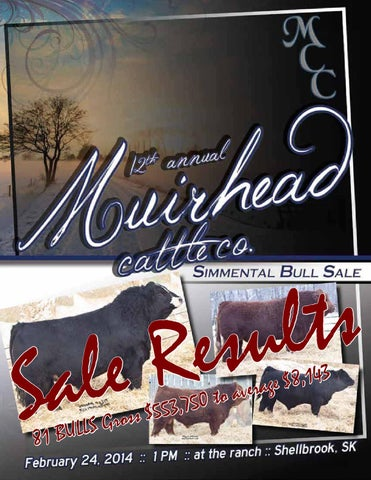l a S

s t l u s e R e

81

s o r G BULLS

a o t 0 5 7 , 3 5 s $5 3 4 1 , 8$ e g a r e v

$9,250$9,500

$8,500$9,500

$6,750$9,000

$8,500$9,000

$7,000$5,250

$5,250$10,500

$5,750$9,000

Out of Sale

$8,750$9,000

$9,000$5,750

$8,750$8,750

$85,000 Labatte &amp; Circle 7$5,000

$7,500$5,500

$9,750$9,750

$5,500$7,000

$9,750$8,750

$5,250 Out of Sale$9,000

$7,250 Out of Sale Out of Sale$6,250

$5,000$6,500

$6,500$7,500

$3,250$8,000

$3,000$3,000

$3,250$9,000

$6,750$9,500

$9,000 Out of Sale Out of Sale$5,000

$4,500$9,000

$7,500$7,250

Out of Sale

$6,750$5,500

$7,250$2,750

$3,250$9,250

$7,000$4,250

$4,250$5,750

$7,500$8,000

$4,000$5,500

Out of Sale

ADDED LOTS Lot 75 $5,250 Lot 76$7,250